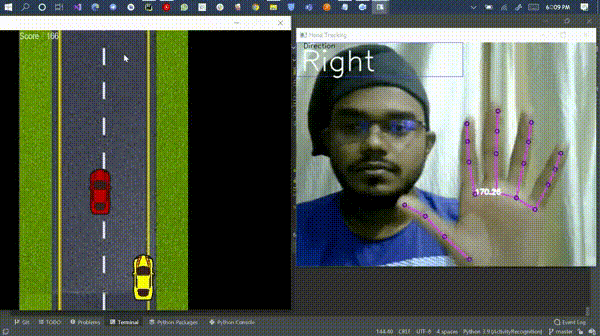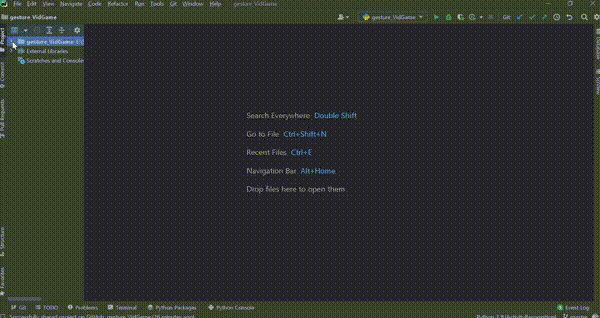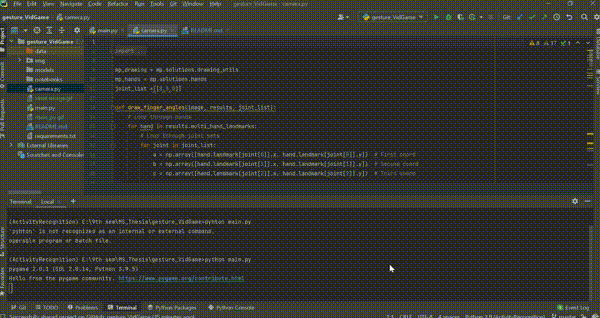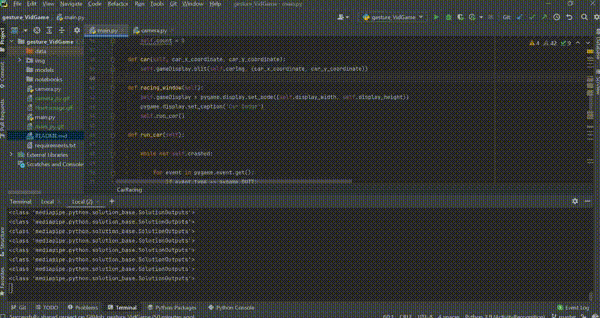aslananuragi — June 16, 2021

This article was published as a part of the Data Science Blogathon

### Want to develop a fantastic game like shown below??## Introduction

The basic idea of this game is First to develop a Hand pose Estimation program and then create a simple Car Doge Game. After that, Communicate between both of the programs by simulating Keyboard’s keypress.

### Prerequisite

• Basic Knowledge of Python, OpenCV, PyGame
• Usage of PyCharm (Optional)

Let’s dig in

## Creating Hand Pose Estimation Application

#### Import the Libraries

```import mediapipe as mp
import cv2
import numpy as np
import uuid
import os
from pynput.keyboard import Key, Controller```

We use mediapipe primarily for tracking the different joints on our palms. I guess you know why we need cv2 and NumPy? We are going to use pynput.Keyboard for simulating the left and right keypress

### A bit of Theory

The above image shows different landmarks which the MediaPipe Library is tracking. In our case, we will use Landmark 8, 5, and 0, i.e., INDEX_FINGER_TIP, INDEX_FINGER_MCP, and WRIST, respectively. We will calculate the angle between these landmarks, and based on that angle, and you can play the game. Interesting no? Wanna know how you can do that? Let’s see it.

#### Declaring some global variable

```mp_drawing = mp.solutions.drawing_utils # used to draw real-time visuals
mp_hands = mp.solutions.hands           # used to track Hand Landmarks
joint_list =[[8,5,0]]                   # Landmark joint```

#### Finding the angle between the required Landmark

```def draw_finger_angles(image, results, joint_list):
# Loop through hands
for hand in results.multi_hand_landmarks:
# Loop through joint sets
for joint in joint_list:
a = np.array([hand.landmark[joint].x, hand.landmark[joint].y])  # First coord
b = np.array([hand.landmark[joint].x, hand.landmark[joint].y])  # Second coord
c = np.array([hand.landmark[joint].x, hand.landmark[joint].y])  # Third coord
radians = np.arctan2(c - b, c - b) - np.arctan2(a - b, a - b)
angle = np.abs(radians * 180.0 / np.pi)
cv2.putText(image, str(round(angle, 2)), tuple(np.multiply(b, [640, 480]).astype(int)),
cv2.FONT_HERSHEY_SIMPLEX, 0.5, (255, 255, 255), 2, cv2.LINE_AA)
return image, angle```

In the above function, we loop through all the joints set and extract all landmarks’ x and y coordinates.

• hand.landmark[joint].x gives us the x coordinate of the first landmark, in our case, x-coordinate of landmark 8
• hand.landmark[joint].y gives us the y coordinate of the first landmark, in our case, y coordinate of landmark 8
• Similarly, we extract coordinate of other landmarks, i.e., 5 and 0

The following line of code calculates the angle in radian and then converting it to the degree. We convert it to a degree because the angle in degree makes more sense to humans. Then using putText() method of OpenCV, we will display the angle beside the “b” joint that Landmark 5. At last, we return the processed Image and the calculated angle.

## Bonus Part

The following code helps you two classify between the left hand and the right hand. it’s not mandatory to do this for this project, But if you want to explore a bit more than the rest, you can try this

```def get_label(index, hand, results):
output = None
for idx, classification in enumerate(results.multi_handedness):
if classification.classification.index == index:
# Process results
label = classification.classification.label
score = classification.classification.score
text = '{} {}'.format(label, round(score, 2))```
```# Extract Coordinates
coords = tuple(np.multiply(
np.array((hand.landmark[mp_hands.HandLandmark.WRIST].x, hand.landmark[mp_hands.HandLandmark.WRIST].y)),
[640, 480]).astype(int))
output = text, coords
return output```

Summary of the above code checks the number of hands in the image, gives us a confidence score based on the prediction, and then shows LEFT or RIGHT text beside the wrist landmark.

## Visualizing the hand pose Estimation

`cap = cv2.VideoCapture(0)`
```with mp_hands.Hands(min_detection_confidence=0.8, min_tracking_confidence=0.5) as hands:
while cap.isOpened():

# BGR 2 RGB
image = cv2.cvtColor(frame, cv2.COLOR_BGR2RGB)

# Flip on horizontal
image = cv2.flip(image, 1)

# Set flag
image.flags.writeable = False

# Detections
results = hands.process(image)

# Set flag to true
image.flags.writeable = True

# RGB 2 BGR
image = cv2.cvtColor(image, cv2.COLOR_RGB2BGR)

# Detections
print(results)

# Rendering results
if results.multi_hand_landmarks:
for num, hand in enumerate(results.multi_hand_landmarks):
mp_drawing.draw_landmarks(image, hand, mp_hands.HAND_CONNECTIONS,
)

# Render left or right detection
if get_label(num, hand, results):
text, coord = get_label(num, hand, results)
cv2.putText(image, text, coord, cv2.FONT_HERSHEY_SIMPLEX, 1, (255, 255, 255), 2, cv2.LINE_AA)

# Draw angles to image from joint list
image, angle = draw_finger_angles(image, results, joint_list)
keyboard = Controller()
if angle<=180:
keyboard.press(Key.right)
keyboard.release(Key.right)
else:
keyboard.press(Key.left)
keyboard.release(Key.left)

# Save our image
# cv2.imwrite(os.path.join('Output Images', '{}.jpg'.format(uuid.uuid1())), image)
cv2.rectangle(image, (0, 0), (355, 73), (214, 44, 53))
cv2.putText(image, 'Direction', (15, 12),
cv2.FONT_HERSHEY_SIMPLEX, 0.5, (0, 0, 0), 1, cv2.LINE_AA)
cv2.putText(image, "Left" if angle >180 else "Right",
(10, 60),
cv2.FONT_HERSHEY_SIMPLEX, 2, (255, 255, 255), 2, cv2.LINE_AA)
cv2.imshow('Hand Tracking', image)

if cv2.waitKey(10) & 0xFF == ord('q'):
break

cap.release()
cv2.destroyAllWindows()```

In the above code line, no.1 will use your webcam to get the camera feed. Then we have a loop that filters out the hand detection only with the min_detection_confidence of 80%, min_tracking_confidence of 50%. Inside the loop, first, we convert the color-coding of the image from BGR to RGB. We do this because OpenCV read image in BRG whereas MediaPipe requires RGB to process it. We also flip the Image because Images captured by the webcam are latterly inverted. Then in Line no. 17, we get the results processed by the MediaPipe. And then, we convert our processed image back to BGR.

## Rendering Results

Between Line no. 28 and 39, we actually display the Lines segment joining the different Landmarks with the color you want.

Further, we call our draw_finger_angle() a function and get the resulting angle made by the landmark we have chosen Earlier. Based on this angle, we move the car left and right. If the angle is less than 180, then virtually press the right arrow key, i.e., drive the car to the right. Otherwise, move the car to the left by virtually pressing the left key.

At last display, some text on the window based on the angle you get and close the loop.

Hurray, you just have completed 70% of the task. Give yourself some appreciation. Now have some coffee and come back to complete the rest of the work.

Great Work !!!

## Developing a Simple Car Dodge Game

```import random            # For placing enemy car Randomal
from time import sleep   #For Debugging
import pygame            # Main Library for creating the game```

I created a CarRacing class for the whole game. Download the images required for the game from here

```import mediapipe as mp
import cv2
import numpy as np
import uuid
import os```
```mp_drawing = mp.solutions.drawing_utils
mp_hands = mp.solutions.hands

import random
from time import sleep
import pygame

class CarRacing:
def __init__(self):

pygame.init()
#pygame.camera.init()
self.display_width = 800
self.display_height = 600
self.black = (0, 0, 0)
self.white = (255, 255, 255)
self.clock = pygame.time.Clock()
self.gameDisplay = None

self.initialize()

def initialize(self):

self.crashed = False

self.car_x_coordinate = (self.display_width * 0.45)
self.car_y_coordinate = (self.display_height * 0.8)
self.car_width = 49

# enemy_car
self.enemy_car_startx = random.randrange(310, 450)
self.enemy_car_starty = -600
self.enemy_car_speed = 5
self.enemy_car_width = 49
self.enemy_car_height = 100

# Background
self.bg_x1 = (self.display_width / 2) - (360 / 2)
self.bg_x2 = (self.display_width / 2) - (360 / 2)
self.bg_y1 = 0
self.bg_y2 = -600
self.bg_speed = 3
self.count = 0

def car(self, car_x_coordinate, car_y_coordinate):
self.gameDisplay.blit(self.carImg, (car_x_coordinate, car_y_coordinate))

def racing_window(self):
self.gameDisplay = pygame.display.set_mode((self.display_width, self.display_height))
pygame.display.set_caption('Car Dodge')
self.run_car()

def run_car(self):

while not self.crashed:

for event in pygame.event.get():
if event.type == pygame.QUIT:
self.crashed = True
# print(event)

if (event.type == pygame.KEYDOWN):
if (event.key == pygame.K_LEFT):
if (self.car_x_coordinate>=340):
self.car_x_coordinate -= 50
print ("CAR X COORDINATES: %s" % self.car_x_coordinate)
if (event.key == pygame.K_RIGHT):
if (self.car_x_coordinate < 440):
self.car_x_coordinate += 50
print ("CAR X COORDINATES: %s" % self.car_x_coordinate)
print ("x: {x}, y: {y}".format(x=self.car_x_coordinate, y=self.car_y_coordinate))

self.gameDisplay.fill(self.black)
self.back_ground_raod()

self.run_enemy_car(self.enemy_car_startx, self.enemy_car_starty)
self.enemy_car_starty += self.enemy_car_speed

if self.enemy_car_starty > self.display_height:
self.enemy_car_starty = 0 - self.enemy_car_height
self.enemy_car_startx = random.randrange(310, 450)

self.car(self.car_x_coordinate, self.car_y_coordinate)
self.highscore(self.count)
self.count += 1
if (self.count % 100 == 0):
self.enemy_car_speed += 1
self.bg_speed += 1
if self.car_y_coordinate < self.enemy_car_starty + self.enemy_car_height:
if self.car_x_coordinate > self.enemy_car_startx and self.car_x_coordinate < self.enemy_car_startx + self.enemy_car_width or self.car_x_coordinate + self.car_width > self.enemy_car_startx and self.car_x_coordinate + self.car_width < self.enemy_car_startx + self.enemy_car_width:
self.crashed = True
self.display_message("Game Over !!!")

if self.car_x_coordinate < 310 or self.car_x_coordinate > 460:
self.crashed = True
self.display_message("Game Over !!!")

pygame.display.update()
self.clock.tick(60)

def display_message(self, msg):
font = pygame.font.SysFont("comicsansms", 72, True)
text = font.render(msg, True, (255, 255, 255))
self.gameDisplay.blit(text, (400 - text.get_width() // 2, 240 - text.get_height() // 2))
self.display_credit()
pygame.display.update()
self.clock.tick(60)
sleep(1)
car_racing.initialize()
car_racing.racing_window()

def back_ground_raod(self):
self.gameDisplay.blit(self.bgImg, (self.bg_x1, self.bg_y1))
self.gameDisplay.blit(self.bgImg, (self.bg_x2, self.bg_y2))

self.bg_y1 += self.bg_speed
self.bg_y2 += self.bg_speed

if self.bg_y1 >= self.display_height:
self.bg_y1 = -600

if self.bg_y2 >= self.display_height:
self.bg_y2 = -600

def run_enemy_car(self, thingx, thingy):
self.gameDisplay.blit(self.enemy_car, (thingx, thingy))

def highscore(self, count):
font = pygame.font.SysFont("arial", 20)
text = font.render("Score : " + str(count), True, self.white)
self.gameDisplay.blit(text, (220, 0))

def display_credit(self):
font = pygame.font.SysFont("lucidaconsole", 14)
text = font.render("Thanks for playing!", True, self.white)
self.gameDisplay.blit(text, (600, 520))

car_racing = CarRacing()
car_racing.racing_window()
sleep(10)```
• __init__(): Initialize the pygame, create a window with the given parameter.
• initialize():  In this, we positioned the enemy and players’ cars on the map.
• car(): Display our car on the game window
• run_car(): As the name suggests, it actually runs the car. In a nutshell, if the left key is pressed, the car shifts to the left by 50 units on the x-axis. If the right key is pressed, the car shifts to the right by 50 units on the x-axis. Now part of it sees whether the car is collied with the enemy car or not by checking the current position of the enemy car and the player’s car position on the x-axis. If it collides, It shows “GAME OVER” and restarts the game.

The other functions are elementary and explain themselves by their name.

### Now you are ready to play the game!!!

Now open a terminal in the current directory and run `python main.py.` Remember, Don’t Close the game Window. Only minimize itNow Open Another terminal in the same directory and run ‘python camera.py‘ Remember Don’t Close This Window, only to minimize it, both the window needs to be running simultaneously.This is the last step, but the most important step. If you have followed me till now, you have got two different windows one which shows the camera feed, and the one which shows the game. Now place these two windows side by side and click on the game window. If you don’t click on the game window, your camera feed will freeze. Note In the following gif, when my cursor is on the hand tracking window, the camera feed freezes. So to avoid it move your cursor and click on the game window.### Congratulations, You did it. You created the Gesture Controlled Video Game.

Are you facing Trouble? Need an organized Code? head to my

Want to connect/collaborate with me? Follow me on and .

The media shown in this article are not owned by Analytics Vidhya and are used at the Author’s discretion.